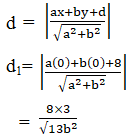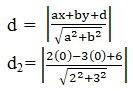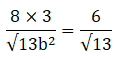• +91 9971497814
• info@interviewmaterial.com

# RD Chapter 23- The Straight Lines Ex-23.6 Interview Questions Answers

### Related Subjects

Question 1 :
Find the equation to the straight line
(i) cutting off intercepts 3 and 2 from the axes.
(ii) cutting off intercepts -5 and 6 from the axes.

(i) Cutting off intercepts3 and 2 from the axes.

Given:

a = 3, b = 2

Let us find theequation of line cutoff intercepts from the axes.

By using the formula,

The equation of theline is x/a + y/b = 1

x/3 + y/2 = 1

By taking LCM,

2x + 3y = 6

The equation of linecut off intercepts 3 and 2 from the axes is 2x + 3y = 6

(ii) Cutting off intercepts-5 and 6 from the axes.

Given:

a = -5, b = 6

Let us find theequation of line cutoff intercepts from the axes.

By using the formula,

The equation of theline is x/a + y/b = 1

x/-5 + y/6 = 1

By taking LCM,

6x – 5y = -30

The equation of linecut off intercepts 3 and 2 from the axes is 6x – 5y = -30

Question 2 :

Find the equation of the straight line which passes through (1, -2) andcuts off equal intercepts on the axes.

Given:

A line passingthrough (1, -2)

Let us assume, theequation of the line cutting equal intercepts at coordinates of length ‘a’ is

By using the formula,

The equation of theline is x/a + y/b = 1

x/a + y/a = 1

x + y = a

The line x + y = apasses through (1, -2)

Hence, the pointsatisfies the equation.

1 -2 = a

a = -1

The equation of theline is x+ y = -1

Question 3 :

Find the equation to the straight line which passes through the point (5,6) and has intercepts on the axes
(i) Equal in magnitude and both positive

(ii) Equal in magnitude but opposite in sign

(i) Equal in magnitude andboth positive

Given:

a = b

Let us find theequation of line cutoff intercepts from the axes.

By using the formula,

The equation of theline is x/a + y/b = 1

x/a + y/a = 1

x + y = a

The line passesthrough the point (5, 6)

Hence, the equationsatisfies the points.

5 + 6 = a

a = 11

The equation of theline is x + y = 11

(ii) Equal in magnitude butopposite in sign

Given:

b = -a

Let us find theequation of line cutoff intercepts from the axes.

By using the formula,

The equation of theline is x/a + y/b = 1

x/a + y/-a = 1

x – y = a

The line passesthrough the point (5, 6)

Hence, the equationsatisfies the points.

5 – 6 = a

a = -1

The equation of theline is x – y = -1

Question 4 :

For what values of a and b the intercepts cut off on the coordinate axesby the line ax + by + 8 = 0 are equal in length but opposite in signs to thosecut off by the line 2x – 3y + 6 = 0 on the axes.

Given:

Intercepts cut off onthe coordinate axes by the line ax + by +8 = 0 …… (i)

And are equal inlength but opposite in sign to those cut off by the line

2x – 3y +6 = 0 ……(ii)

We know that, theslope of two lines is equal

The slope of theline (i) is –a/b

The slope of theline (ii) is 2/3

So let us equate,

-a/b = 2/3

a = -2b/3

The length of theperpendicular from the origin to the line (i) is

By using the formula,The length of theperpendicular from the origin to the line (ii) is

By using the formula,It is given that, d1 =d2b = 4

So, a = -2b/3

= -8/3

The value of a is-8/3 and b is 4.

Question 5 : Find the equation to the straight line which cuts off equal positive intercepts on the axes and their product is 25.

Given:

a = b and ab = 25

Let us find theequation of the line which cutoff intercepts on the axes.

a2 =25

a = 5 [consideringonly positive value of intercepts]

By using the formula,

The equation of theline with intercepts a and b is x/a + y/b = 1

x/5 + y/5 = 1

By taking LCM

x + y = 5

The equation of lineis x + y = 5

Todays Deals### RD Chapter 23- The Straight Lines Ex-23.6 Contributorskrishan

Name:
Email:

# Latest News# 9000 interview questions in different categories